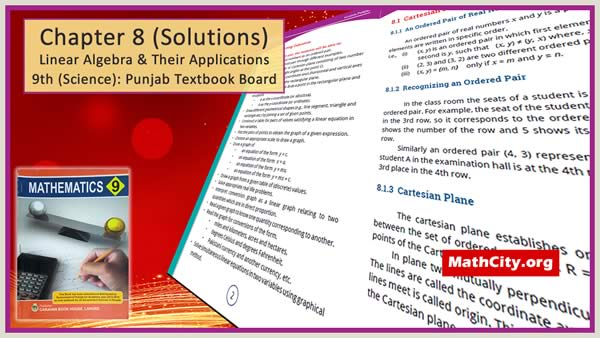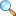# Unit 08: Linear Graph and their Application

On this page notes of Unit 08 of Mathematics 9 written by Dr. Karamat H. Dar and Prof. Irfan-ul-Haq are given.After studying this unit the students will be able to:

• Identity pair of real numbers as an ordered pair.
• Recognize an ordered pair through different examples.
• Describe rectangular or cartesian plane consisting of two number lines interesting at right angles at the point $O$ .
• Identify origin $\left( O \right)$ and coordinate axes (horizontal and vertical axes or x-axis and y-axis) in the rectangular plane.
• Locate an ordered pair $\left( a,b \right)$ as a point in the rectangular plane and recognize.
• $a\,$ as the x-coordinates (or abscissa).
• $b$ as the y-coordinate (or ordinate).
• Draw different geometrical shapes (e.g., line segment, triangle and rectangle etc.) by joining a set of given points.
• Construct a table for pairs of values satisfying a linear equation in two variables.
• Plot the pairs of points to obtain the graph of a given expression.
• Chose an appropriate scale to draw a graph.
• Draw the graph of
• an equation of the form $y=c.$
• an equation f the form $x=a.$
• an equation f the form $y=mx.$
• an equation f the form $y=mx+c.$
• Draw the graph from the given table of (discrete) values.
• Solve appropriate real life problems.
• Interpret conversion graph as a linear graph relating to two equations which are in direct proportion
• Read the graph to know one quantity corresponding to another.
• Read the graph of conversions of the fom.
• miles and kilometers,
• acres and hectares,
• degrees Celsius and degree Fahrenheit,
• Pakistani currency and another currency, etc.
• Solve simultaneous linear equations in two variables using graphical method.
• Exercise 8.2 |View Online | Download PDF• Exercise 8.3 |View Online | Download PDF• matric/9th_science/unit08